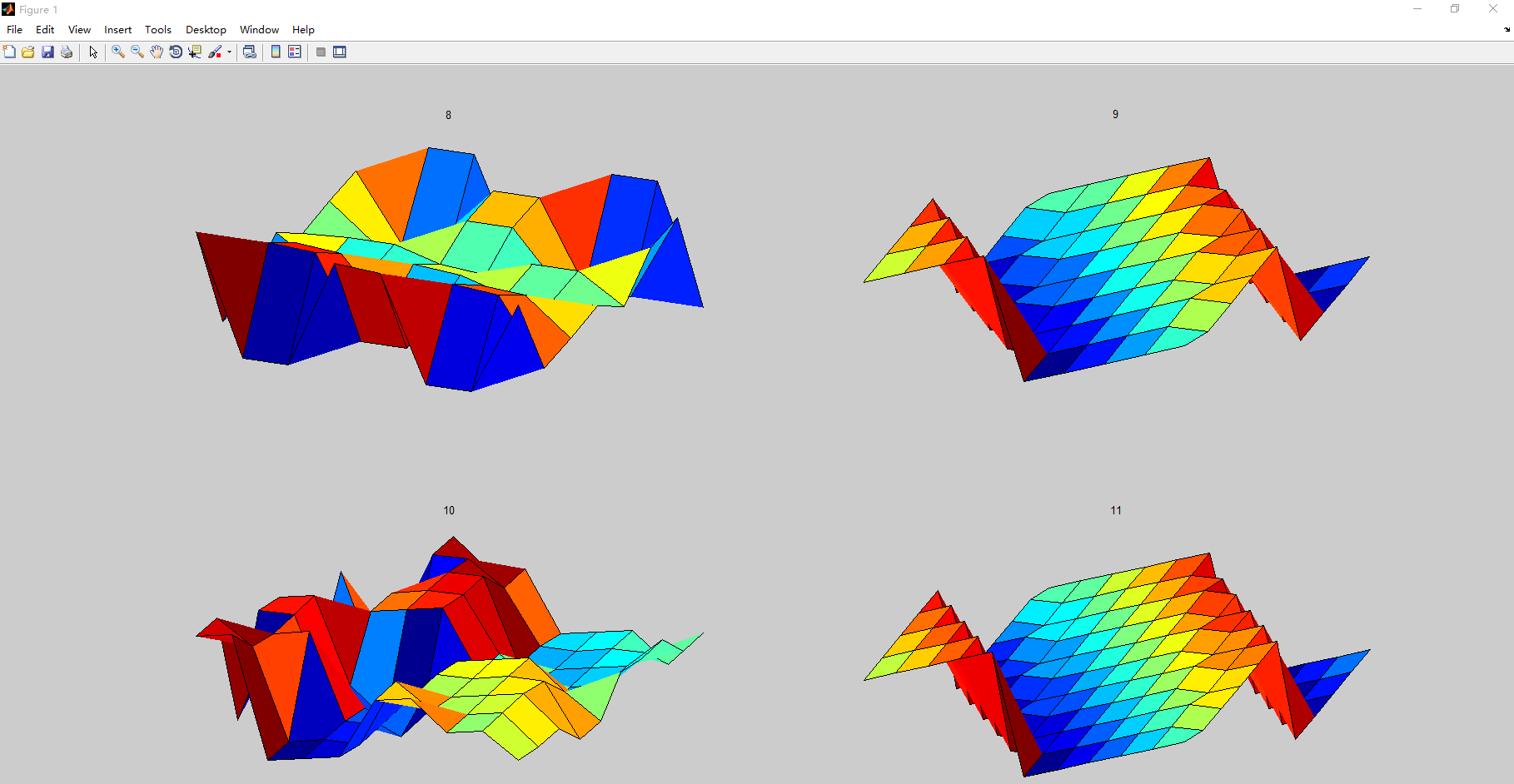# matlab magic

2015/10/26 14:11

for n = 8:11
subplot(2,2,n-7)
surf(magic(n))
title(num2str(n))
axis off
view(30,45)
axis tight
endfunction M = magicV2(n)

%magicV2  magicV2 square.

%   magicV2(N) is an N-by-N matrix constructed from the integers

%   1 through N^2 with equal row, column, and diagonal sums.

%   Produces valid magicV2 squares for all N > 0 except N = 2.

%   Copyright 1984-2002 The MathWorks, Inc.

%   $Revision: 5.15$  $Date: 2002/04/15 03:44:23$

% Historically, MATLAB's magicV2 was a built-in function.

% This M-file uses a new algorithm to generate the same matrices.

n = floor(real(double(n(1))));

% Odd order.

if mod(n,2) == 1

[J,I] = meshgrid(1:n);

A = mod(I+J-(n+3)/2,n);

B = mod(I+2*J-2,n);

M = n*A + B + 1;

% Doubly even order.

elseif mod(n,4) == 0

[J,I] = meshgrid(1:n);

K = fix(mod(I,4)/2) == fix(mod(J,4)/2);

M = reshape(1:n*n,n,n)';

M(K) = n*n+1 - M(K);

% Singly even order.

else

p = n/2;

M = magicV2(p);

M = [M M+2*p^2; M+3*p^2 M+p^2];

if n == 2, return, end

i = (1:p)';

k = (n-2)/4;

j = [1:k (n-k+2):n];

M([i; i+p],j) = M([i+p; i],j);

i = k+1;

j = [1 i];

M([i; i+p],j) = M([i+p; i],j);

end

0
0 收藏

### 作者的其它热门文章This MATLAB function uses variable-precision floating-point arithmetic (VPA) to evaluate each element of the symbolic input x to at least d significant digits
2015/11/02 14:48

1 评论
0 收藏
0